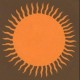## Atiyah lecture at 2018 Heidelberg Laureate ForumI have an earlier blogpost from 2 years ago titled Atiyah lecture at 2016 Heildelberg Laureate Forum.

Atiyah gave another lecture at the Heidelberg Laurate Forum this year. His lecture created great excitement. He presented a proof of the Riemann Hypothesis. It is amazing that at the age of 89 he has the energy, ambition and the sharpness of the mind to make an attempt to prove the Riemann Hypothesis. Truly amazing!

In the abstract of his lecture he says “The Riemann Hypothesis is a famous unsolved problem dating from 1859. I will present a simple proof using a radically new approach. It is based on work of von Neumann (1936), Hirzebruch (1954) and Dirac (1928).

Atiyah lecture at 2018 Heidelberg Laureate Forum (video)

He also provided 2 papers on his proof of the Riemann Hypothesis.

The Fine Structure Constant

The Riemann Hypothesis

Frankie Schembri’s article at the Science magazine informs us that mathematicians are skeptical about Atiyah’s proof of the Riemann’s Hypothesis. Here’s few quotes from Schembri’s article:

“The Riemann hypothesis, one of the last great unsolved problems in math, was first proposed in 1859 by German mathematician Bernhard Riemann. It is a supposition about prime numbers, such as two, three, five, seven, and 11, which can only be divided by one or themselves. They become less frequent, separated by ever-more-distant gaps on the number line. Riemann found that the key to understanding their distribution lay within another set of numbers, the zeroes of a function called the Riemann zeta function that has both real and imaginary inputs. And he invented a formula for calculating how many primes there are, up to a cutoff, and at what intervals these primes occur, based on the zeroes of the zeta function. However, Riemann’s formula only holds if one assumes that the real parts of these zeta function zeroes are all equal to one-half. Reimann proved this property for the first few primes, and over the past century it has been computationally shown to work for many large numbers of primes, but it remains to be formally and indisputably proved out to infinity. A proof would not only win the \$1 million reward that comes for solving one of the seven Millennium Prize Problems established by the Clay Mathematics Institute in 2000, but it could also have applications in predicting prime numbers, important in cryptography.”

“The crux of Atiyah’s proof depends on a quantity in physics called the fine structure constant, which describes the strength and nature of electromagnetic interaction between charged particles. By describing this constant using a relatively obscure relationship known as the Todd function, Atiyah claimed to be able to prove the Riemann hypothesis by contradiction.”